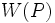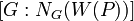# Weakly closed conjugacy functor

(diff) ← Older revision | Latest revision (diff) | Newer revision → (diff)
Jump to: navigation, search
This article defines a property that can be evaluated for a conjugacy functor on a finite group. |View all such properties

## Definition

Suppose$G$ is a finite group,$p$ a prime number, and$W$ a conjugacy functor on$G$ with respect to$p$. We say that$W$ is weakly closed in$G$ with respect to$p$ if the following equivalent conditions are satisfied:

1. Either of these equivalent:
• There exists a$p$-Sylow subgroup$P$ of$G$ such that$W(P)$ is a weakly closed subgroup of$P$ relative to$G$.
• For every$p$-Sylow subgroup$P$ of$G$,$W(P)$ is a weakly closed subgroup of$P$ relative to$G$.
2. Either of these equivalent:
• There exists a$p$-Sylow subgroup$P$ such that, for every$p$-Sylow subgroup$Q$ containing$W(P)$,$W(P) = W(Q)$.
• For every$p$-Sylow subgroup$P$, and for every$p$-Sylow subgroup$Q$ containing$W(P)$,$W(P) = W(Q)$.
3. Either of these equivalent:
• There exists a$p$-Sylow subgroup$P$ of$G$ such that for any$p$-Sylow subgroup$Q$ of$G$ containing$W(P)$,$W(P)$ is a normal subgroup of$Q$.
• For every$p$-Sylow subgroup$P$ of$G$, it is true that for any$p$-Sylow subgroup$Q$ of$G$ containing$W(P)$,$W(P)$ is a normal subgroup of$Q$.

For instance, a p-normal group is a group in which the conjugacy functor that arises by taking the center is weakly closed.

### Equivalence of definitions

Further information: equivalence of definitions of weakly closed conjugacy functor

## Equivalence relation induced on the set of Sylow subgroups

Given a weakly closed conjugacy functor$W$ for a prime$p$, we obtain an equivalence relation on the set$\operatorname{Syl}_p(G)$ of all$p$-Sylow subgroups of$G$. The equivalence relation is as follows: two$p$-Sylow subgroups$P,Q$ are equivalent if they satisfy the above equivalent conditions, for instance,$W(P) = W(Q)$ (this is the equivalent formulation that makes it easiest to see that the relation is reflexive, symmetric, and transitive).

This equivalence relation partitions the set$\operatorname{Syl}_p(G)$ into equivalence classes. It further turns out that all equivalence classes have the same size, because the conjugation with$G$ permutes them transitively. Moreover:

• The equivalence classes are parametrized by the conjugacy class of$W(P)$. The number of such equivalence classes is$[G:N_G(W(P))]$ and the size of each equivalence class is$[N_G(W(P)):N_G(P)]$.
• The equivalence class corresponding to a particular$W = W(P)$ is characterized as precisely those$p$-Sylow subgroups of$G$ that contain$W$.
• By the congruence condition on index of subgroup containing Sylow-normalizer, both the number of orbits and the size of each orbit are congruent to 1 modulo$p$.

Two extreme cases are of interest:

• The case that the equivalence relation has only one equivalence class, which means that$W(P) = W(Q)$ for all$P,Q \in \operatorname{Syl}_p(G)$, or equivalently, the subgroup$W(P)$ is inside$O_p(G)$, the p-core. This is equivalent to$W(P)$ being a normal subgroup of$G$. For more, see conjugacy functor that gives a normal subgroup.
• The case that the equivalence relation has equivalence classes all of size one, i.e., this is the case that$N_G(W(P)) = N_G(P)$.

## Relation with other properties

### Stronger properties

Property Meaning Proof of implication Proof of strictness (reverse implication failure) Intermediate notions
conjugacy functor that gives a normal subgroup Strongly closed conjugacy functor|FULL LIST, MORE INFO
strongly closed conjugacy functor |FULL LIST, MORE INFO